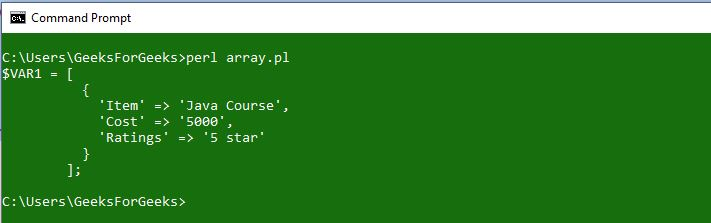Open In App

# Perl | Multidimensional Arrays

Multidimensional arrays in Perl are the arrays with more than one dimension. Technically there is no such thing as a multidimensional array in Perl but arrays are used to act as they have more than one dimension. Multi dimensional arrays are represented in the form of rows and columns, also knows as matrix. Each element of an array can be a reference to another array but in syntax, they will appear like a 2-dimensional array.

A multidimensional array can only hold scalar values, they can not hold arrays or hashes.

### Initialization and Declaration of a Multidimensional Array

Given below is the example that makes clear the initialization and declaration of a Multidimensional array.
In this example we will simply initialize the array by @array_name = ([…], […], […]);

## Perl

 `#!/usr/bin/perl``use` `strict;``use` `warnings;` `# Initializing and defining the array``my` `@items` `= ( [``'book'``, ``'pen'``, ``'pencil'``],``              ``[``'Bat'``, ``'Ball'``, ``'Stumps'``],``              ``[``'snake'``, ``'rat'``, ``'rabbit'``] );` `# Printing items from the array``print` `\$items``, ``"\n"``;``print` `\$items``, ``"\n"``;``print` `\$items``, ``"\n"``;`

Output: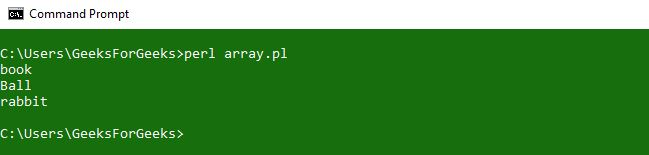### Creating a Matrix

Matrix is a collection of rows and columns of arrays that appears to be in more than one dimension.
Let’s see an example to make it more clear of creating a matrix in Perl.

Example 1:
In this example, we first declared three arrays with values and then merged them into a final resulted array to form a 3*3 matrix. In order to control the variable \$m and \$n, two for loops are used.

## Perl

 `#!/usr/bin/perl``use` `strict;``use` `warnings;` `# Array Declaration``my` `@arrayA` `= ``qw(1 0 0)``; ``my` `@arrayB` `= ``qw(0 1 0)``; ``my` `@arrayC` `= ``qw(0 0 1)``; ` `# Merging 3 arrays into One Final array ``my` `@result` `= (\``@arrayA``, \``@arrayB``, \``@arrayC``);`` ` `print` `"Resultant 3*3 Matrix:\n"``; ` `# Using For loop``for``(``my` `\$m` `= 0; ``\$m` `<= ``\$#result``; ``\$m``++)``{  ``   ``for``(``my` `\$n` `= 0; ``\$n` `<= ``\$#result` `; ``\$n``++)``   ``{ ``      ``print` `"\$result[\$m][\$n] "``; ``   ``} ``   ``print` `"\n"``; ``} `

Output: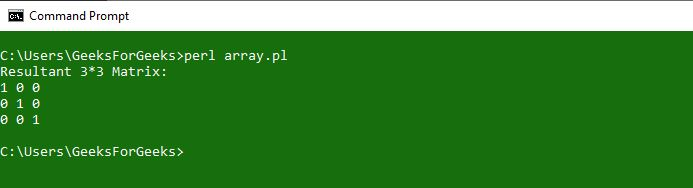Example 2:
In this example, we will take the input from the keyboard and then add the two matrices and printing the result using the resultant matrix.

## Perl

 `#!usr/bin/perl``use` `strict;``use` `warnings;` `my` `(``@MatrixA``, ``@MatrixB``, ``@Resultant``) = ((), (), ());` `# Asking for User Input for Matrix A``print` `"Please Provide the order of MatrixA :\n"``;``print` `"\tMatrixA rows:"``;``chomp``(``my` `\$rowA` `= <>);  ``# CHOMP TO TAKE USER INPUT``print` `"\tMatrixA columns:"``;``chomp``(``my` `\$columnA` `= <>);` `# Asking for User Input for Matrix B``print` `"Please Provide the order of MatrixB :\n"``;``print` `"\tMatrixB rows:"``;``chomp``(``my` `\$rowB` `= <>);``print` `"\tMatrixB columns:"``;``chomp``(``my` `\$columnB` `= <>);` `# Asking User to input elements of matrices``if` `(``\$rowA` `== ``\$rowB` `and ``\$columnA` `== ``\$columnB``)``{``    ``print` `"Enter \$rowA * \$columnA elements in MatrixA:\n"``;``    ``foreach` `my` `\$m` `(0..``\$rowA` `- 1)``    ``{``        ``foreach` `my` `\$n` `(0..``\$columnA` `- 1)``        ``{``            ``chomp``(``\$MatrixA``[``\$m``][``\$n``] = <>);  ``# TO READ THE VALUES``        ``}``    ``}``    ` `    ``print` `"Enter \$rowB * \$columnB elements in MatrixB:\n"``;``    ``foreach` `my` `\$m` `(0..``\$rowB` `- 1)``    ``{``        ``foreach` `my` `\$n` `(0..``\$columnB` `- 1)``        ``{``            ``chomp``(``\$MatrixB``[``\$m``][``\$n``] = <>);  ``# TO READ THE VALUES``        ``}``    ``}``    ` `    ``# Performing Addition operation``    ``foreach` `my` `\$m` `(0..``\$rowB` `- 1)``    ``{``        ``foreach` `my` `\$n` `(0..``\$columnB` `- 1)``        ``{``            ``\$Resultant``[``\$m``][``\$n``] = ``\$MatrixA``[``\$m``][``\$n``] +``                                 ``\$MatrixB``[``\$m``][``\$n``];``        ``}``    ``}``    ` `    ``# Printing Matrix A``    ``print` `"MatrixA is :\n"``;``    ``foreach` `my` `\$m` `(0..``\$rowB` `- 1)``    ``{``        ``foreach` `my` `\$n` `(0..``\$columnB` `- 1)``        ``{``            ``print` `"\$MatrixA[\$m][\$n] "``;``        ``}``        ``print` `"\n"``;``    ``}``    ` `    ``# Printing Matrix B``    ``print` `"MatrixB is :\n"``;``    ``foreach` `my` `\$m` `(0..``\$rowB` `- 1)``    ``{``        ``foreach` `my` `\$n` `(0..``\$columnB` `- 1)``        ``{``            ``print` `"\$MatrixB[\$m][\$n] "``;``        ``}``        ``print` `"\n"``;``    ``}``    ` `    ``# Printing the sum of Matrices``    ``print` `"SUM of MatrixA and MatrixB is :\n"``;``    ``foreach` `my` `\$m` `(0..``\$rowB` `- 1)``    ``{``        ``foreach` `my` `\$n` `(0..``\$columnB` `- 1)``        ``{``            ``print` `"\$Resultant[\$m][\$n] "``;``        ``}``        ``print` `"\n"``;``    ``}``}` `# Error if Matrices are of different order``else``{``    ``print` `"Matrices order does not MATCH, Addition is not Possible"``;``}`

Output: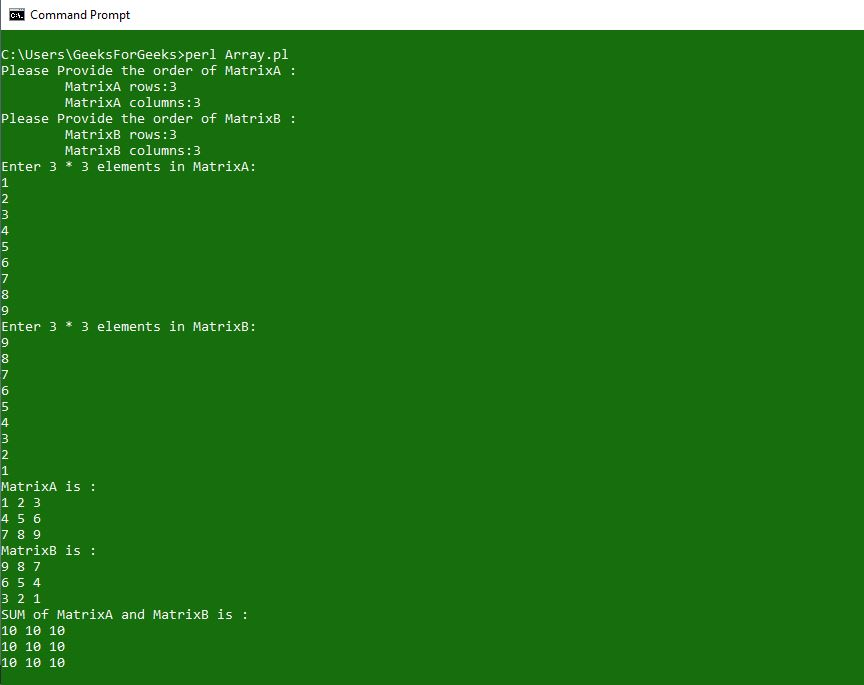Addition of Matrices can only be performed if the matrices are of the same order.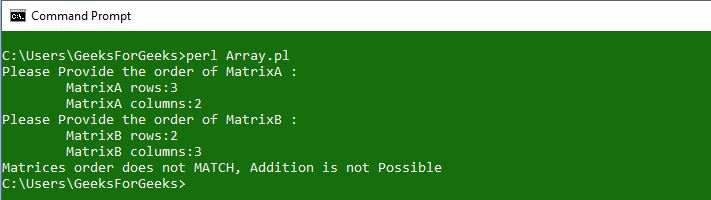### Array of Arrays

Array of Arrays are the data structures having an array having list of array references. Elements inside an array are the array references. These references can be printed individually or the whole array can be printed as well, as per the requirement.

Syntax:

`@ARRAY_NAME = ([value1], [value2], [value3], ..., [valueN]);`

Example 1:

## Perl

 `#!/usr/bin/perl``use` `strict;``use` `warnings;` `# Array of Arrays``my` `@GfG` `= ( [``"Geek"``,  ``"For"``,  ``"Geeks"``],``            ``[``"Is"``,    ``"Best"``, ``"For"``],``            ``[``"Those"``, ``"In"``,  ``"Need"``] );` `# Printing internal array elements``print` `"Accessing Array elements:\n"``;``print`  `@{``\$GfG``}, ``"\n"``; ``# For accessing particular array element``print`  `@{``\$GfG``}, ``"\n"``;``print`  `@{``\$GfG``}, ``"\n"``;` `# Printing whole Arrays``print` `"\nAccessing whole arrays: \n"``;``print` `@{``\$GfG``}, ``"\n"``; ``# For accessing particular array``print` `@{``\$GfG``}, ``"\n"``;``print` `@{``\$GfG``}, ``"\n"``;``print` `"\n"``;`

Output: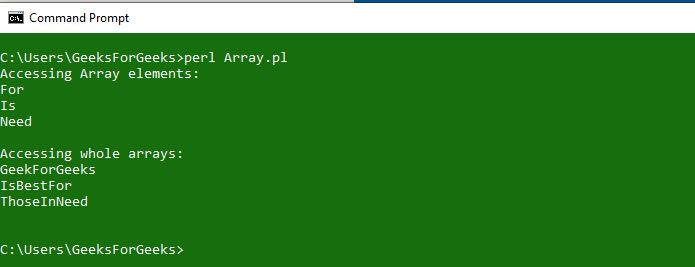Example 2:

In this example, we generated an array of array by using a single array.

## Perl

 `#!/usr/bin/perl``use` `strict;``use` `warnings;``use` `Data::Dumper;` `my` `@GfG` `= ( [``"SAM"``,  ``"SHABANAM"``,  ``"SOHAM"``],``            ``[``"DHONI"``, ``"GONI"``, ``"AVNI"``],``            ``[``"VIRAT"``, ``"STUART"``, ``"ROHIT"``] );``my` `@test``;``foreach` `(``@GfG``)``{``    ``if` `(``\$_` `=~ /M/ )``    ``{``        ``push` `( @{``\$test``}, ``\$_``);``    ``}``    ``elsif` `(``\$_` `=~ /I/ )``    ``{``        ``push` `( @{``\$test``}, ``\$_``);``    ``}``    ``else``    ``{``        ``push` `( @{``\$test``}, ``\$_``);``    ``}``}` `print` `Dumper(\``@test``), ``"\n"``;`

Output: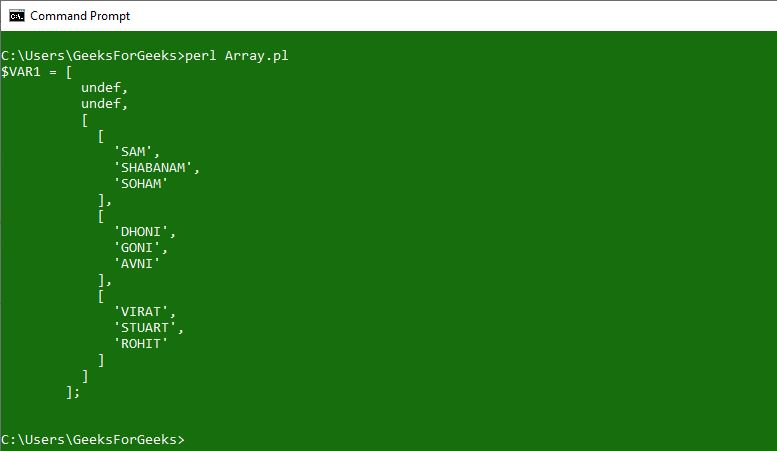### Array of Hashes

Array of hashes is the data structure where the array has a list of hashes. Hash references are the objects of an array. In order to access the key and values, we need to de-reference them.
Array of hashes is a great data structure if we want to loop through hashes numerically.

Syntax:

`@ARRAY_NAME = ({KEY1 => VALUE1}, {KEY2 => VALUE2});`

Example 1:

## Perl

 `#!/usr/bin/perl``use` `strict;``use` `warnings;` `my` `@hashtest` `= ({ ``First` `=> ``"Geek"``,``                  ``Middle` `=> ``"For"``,``                  ``Last` `=> ``"Geeks"` `},``                ``{ ``Street` `=> ``"Royal Kapsons"``,``                  ``City` `=> ``"Noida-Uttar Pradesh"` `},``                ``{ ``About` `=> ``"Computer Science portal"``,``                  ``Content` `=> ``"Technical"` `});` `# To access any of the hash reference.``print` `\$hashtest``, ``"\n"``;` `# To access keys of any hash reference.``print` `keys` `%{``\$hashtest``}, ``"\n"``;` `# To access any value of any key.``print` `\$hashtest``->{``"First"``}, ``"\n"``;``print` `\$hashtest``->{``"Middle"``}, ``"\n"``;``print` `\$hashtest``->{``"Last"``}, ``"\n"``;`

Output: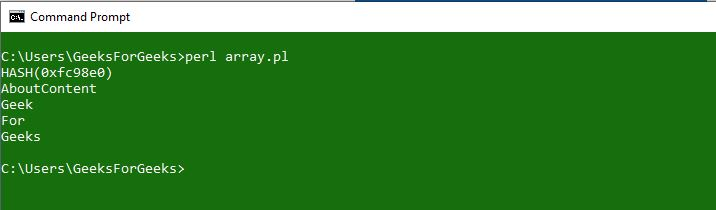Example 2:
Generating an array of hashes from a simple array.

## Perl

 `#!/usr/bin/perl``use` `strict;``use` `warnings;` `use` `Data::Dumper;` `my` `@array` `= (``"Item - Java Course"``,``             ``"Cost - 5000"``,``             ``"Ratings - 5 star"``);` `# Empty array of hashes``my` `@array_1``;` `foreach` `(``@array``)``{``    ``my` `@array` `= ``split``(``" - "``, ``\$_``);``    ``\$array_1``->{``\$array``} = ``\$array``;``}` `print` `Dumper (\``@array_1``);`

Output: